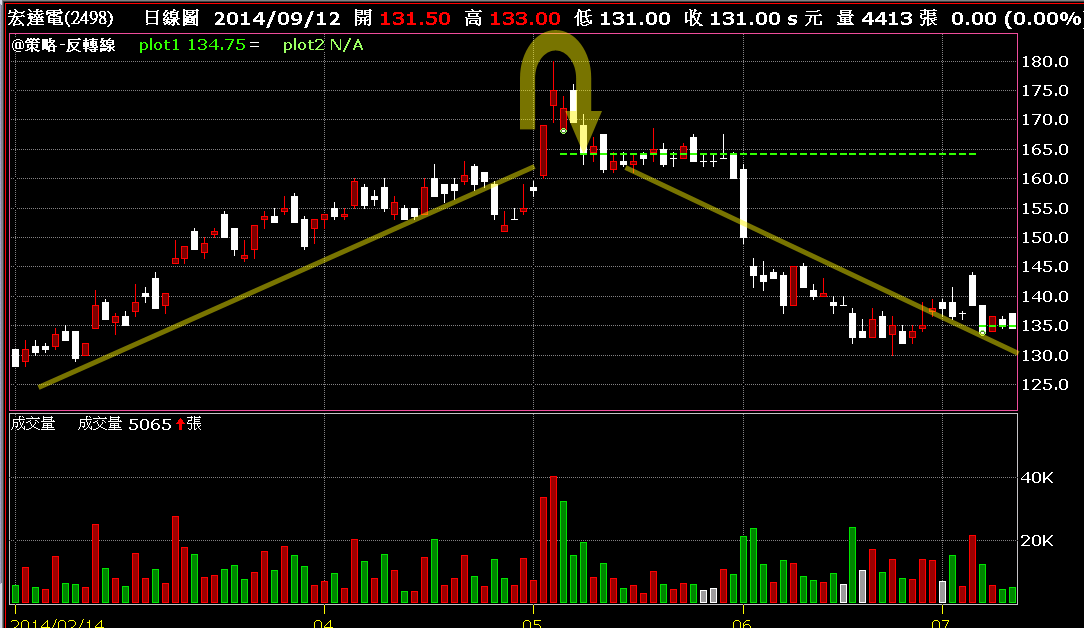# 典型反轉訊號當指數或股價處在高檔時,跑得快是一個很重要的反射機制,跑得對則是一種藝術!

condition1= H > H and H > H and H > L*1.05 and H > L*1.05;

if condition1 then value1 = (L+L)/2;

{警示} if Close cross under value1 then ret=1;

{指標} plot1(Value1,”高檔反轉線”);

``` condition1= H > H and H > H and H > L*1.05 and H > L*1.05; if condition1 then value1 = (L+L)/2; if Close cross under value1 then ret=1; ```

``` condition1= H > H and H > H and H > L*1.05 and H > L*1.05; if condition1 then value1 = (L+L)/2; plot1(Value1,"高檔反轉線"); ```